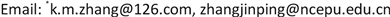1. 引言

1) 通过简单例子说明独立性和条件独立性是互不蕴含的关系。

2) 给出条件独立的判定条件。

2. 主要结果2.1. 独立性和条件独立性

P ( A ∩ B ) = P ( A ) P ( B ) (1)

P ( A ∩ B | C ) = P ( A | C ) P ( B | C ) (2)

B 1 = {第一次取得红球}， B 2 = {第二次取得红球}，

C = {第一次和第二次取得小球的颜色不同}，

R表示红球，W表示白球，则这个随机试验的样本空间为 S = { R W , W R , R R , W W } ，且四种结果是等可能的。

P ( B 1 ) = 1 2 , P ( B 2 ) = 1 2 , P ( B 1 ∩ B 2 ) = 1 4 , P ( B 1 | C ) = 1 2 , P ( B 2 | C ) = 1 2 , P ( B 1 ∩ B 2 | C ) = 0

P ( B 1 ∩ B 2 | C ) ≠ P ( B 1 | C ) P ( B 2 | C ) .

A = { 1 , 2 , 3 , 4 } ,   B = { 2 , 3 , 5 , 7 } ,   C = { 2 , 3 , 7 } .

P ( A ) = 4 9 , P ( B ) = 4 9 , P ( A ∩ B ) = 2 9 , P ( A | C ) = 2 3 , P ( B | C ) = 1 ,   P ( A ∩ B | C ) = 2 3

P ( A ∩ B ) ≠ P ( A ) P ( B ) , P ( A ∩ B | C ) = P ( A | C ) P ( B | C )

2.2. 条件独立性的判定

P ( A | B ∩ C ) = P ( A | C ) (3)

3. 总结